# Introduction

The ability to forecast future events and plan for the future is a uniquely human skill, and a difficult one to master. Through a reframing of one’s memory known as Hindsight Bias,1 an event that was actually very surprising a priori may be perceived to have been predictable by a posteriori. Hence, I have attempted to quantify explicitly a sample of lay-forecasts of seat counts for the major political parties in the 42nd Canadian federal election.

# Methods

Subjective probability distributions were collected using an online collection tool originally developed by Carlisle et al for the STREAM forecasting project.2 Predictors were shown a 20x20 grid, and allowed 40 “chips,” each representing 2.5% certainty, and instructed to place them in bins corresponding to ranges of seats that they predict will be won by each of the major five political parties. For example, if a forecaster placed 10 “chips” in the column labelled “0–16,” that would represent a forecast with 25% certainty that the party in question would receive between 0 and 16 seats in the House of Commons.

The forecast collection tool was available starting 10 days prior to the election, and was closed to new predictions at the time the first polls closed to voting. Forecasters were encouraged to make use of any external polls, articles or predictive models to which they had access, as this was meant to be a test of a person’s ability to produce a well-calibrated forecast of the election, not a test of the person’s memory.

## Exclusions

Forecasts were excluded if they appeared to not represent a good-faith attempt to provide a forecast, or if there appeared to be a fundamental misunderstanding of the forecasting task.

Examples of excluded forecasts include:

• All cells left blank
• All cells filled in
• Alternating filled in and blank columns

In the case of predictions where less than 40 chips were used, the used chips were re-weighted such that the sum of the probabilities of the used chips always equals 1.

Forecasts of the Green Party and the Bloc Quebecois were not included in this analysis. These predictions were excluded because a grid of 20 ranges of approximately 17 seats per column does not allow for a sufficiently granular prediction space. Many forecasters placed 20 chips in the first column (the maximum allowed in a single column) and left the second column blank, while others filled the first two columns with 20 chips each, to use the allotted 40 chips. It was impossible to discern between those who would have used only the first column, had the option been explicitly given, and those who honestly meant to give a prediction that is twice as wide, and so these were excluded for simplicity.

## Statistical methods

Summary probability distributions, $$g$$ over discrete variable $$x$$ (number of seats in the House of Commons) were calculated using the following formula, where $$N$$ is the total number of forecasts, $$w_t$$ is a weighting factor for the forecast in question and $$f_t (x)$$ is the $$t$$-th subjective probability function over $$x$$. Unless otherwise specified, $$w_t = \frac{1}{N}$$

$g(x) = \sum_{t=1}^{N} w_t f_t (x)$

The expectation of a subjective probability distribution was calculated as follows, where $$E$$ is the expectation of some discrete variable $$x$$ (here, the number of seats in the House of Commons), $$N$$ is the number of forecasts, $$f_t (x)$$ is the value of the probability function at $$x$$ for the forecast in question.

$E = \frac{1}{N} \sum_{t=1}^{N} x f_t (x)$

The calibration of a forecast was assessed using Brier scores3 ($$S_B$$), which were calculated using the following formula, in which $$N$$ is the number of forecasts, $$f_t$$ is the value of the probability function for the forecast in question and $$o_t$$ is the outcome for the forecast (0 or 1).

$S_B = \frac{1}{N} \sum_{t=1}^{N} (f_t - o_t)^2$

Differences between groups and hypothesis-testing was done using a two-tailed $$t$$-test using R version 3.2.24 and the scoring package.5

# Results

In total, 87 forecasts were collected between 2015 October 10 and 2015 October 19 at 20:30, Newfoundland time. After applying the above exclusion criteria, 76 were eligible for inclusion.

Sample demographics Total n=76
Preferred party
NDP 26 (34%)
LPC 14 (18%)
GPC 3 (4%)
CPC 2 (3%)
Other 1 (1%)
Not stated 30 (39%)
Party affiliation
NDP 9 (12%)
LPC 3 (4%)
GPC 2 (3%)
CPC 2 (3%)
Other 2 (3%)
Not stated 58 (76%)
Religious affiliation
Christian 10 (13%)
Atheist 13 (17%)
Agnostic 7 (9%)
Other 3 (4%)
Not stated 43 (57%)
Primary news source
CBC 24 (32%)
Internet/Twitter/Reddit 7 (9%)
Globe and Mail 5 (7%)
La Presse 2 (3%)
Mixed 3 (4%)
CTV 1 (1%)
Global 1 (1%)
Le Devoir 1 (1%)
Toronto Star 1 (1%)
Not stated 31 (41%)

## Combined subjective probability distributions

The following are the combined subjective probability distributions, weighted equally among all forecasters. The coloured column represents the range of possible outcomes that includes the actual number of seats won by the party in question, namely 44, 184 and 99 seats for the NDP, LPC and CPC respectively.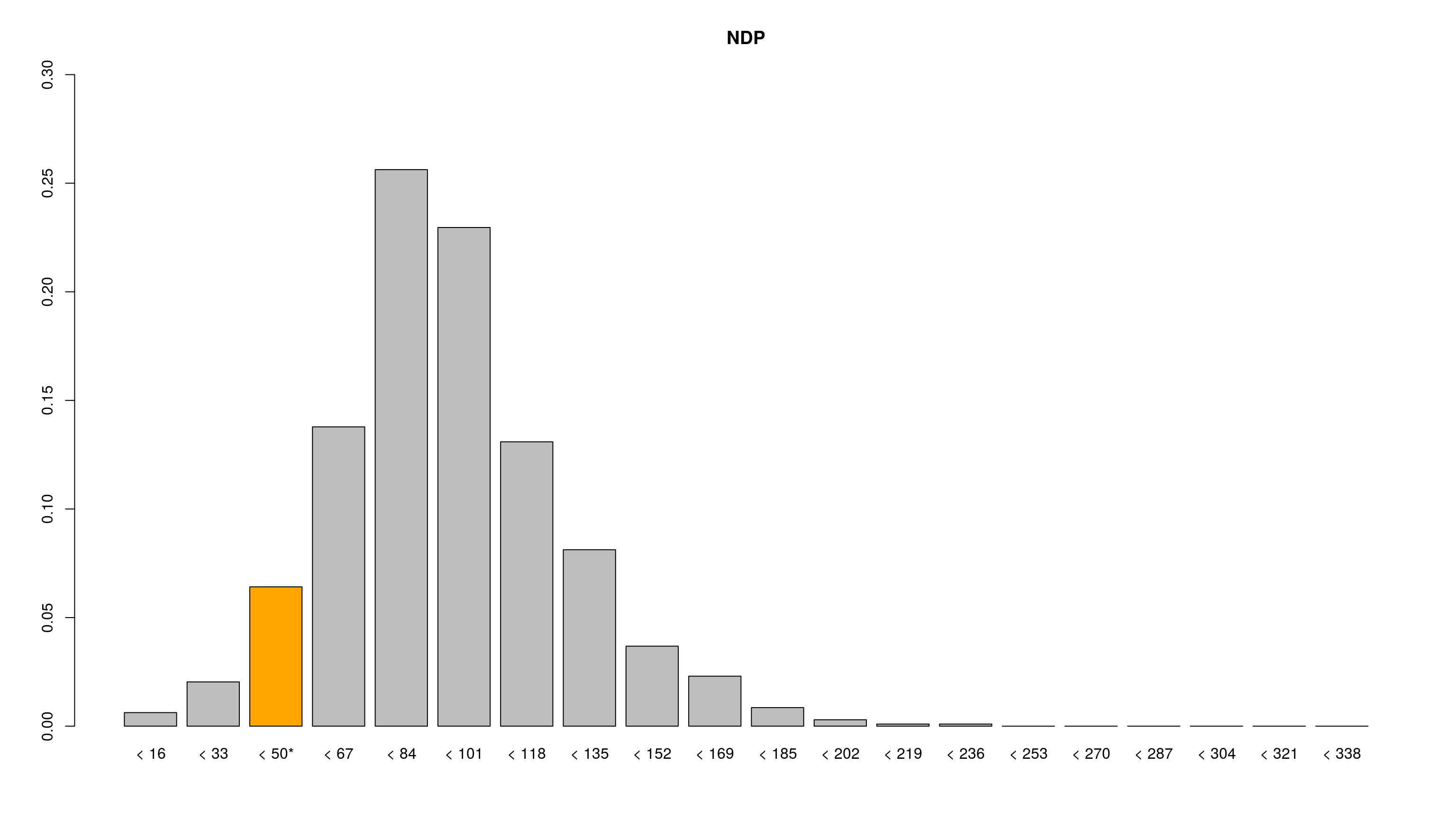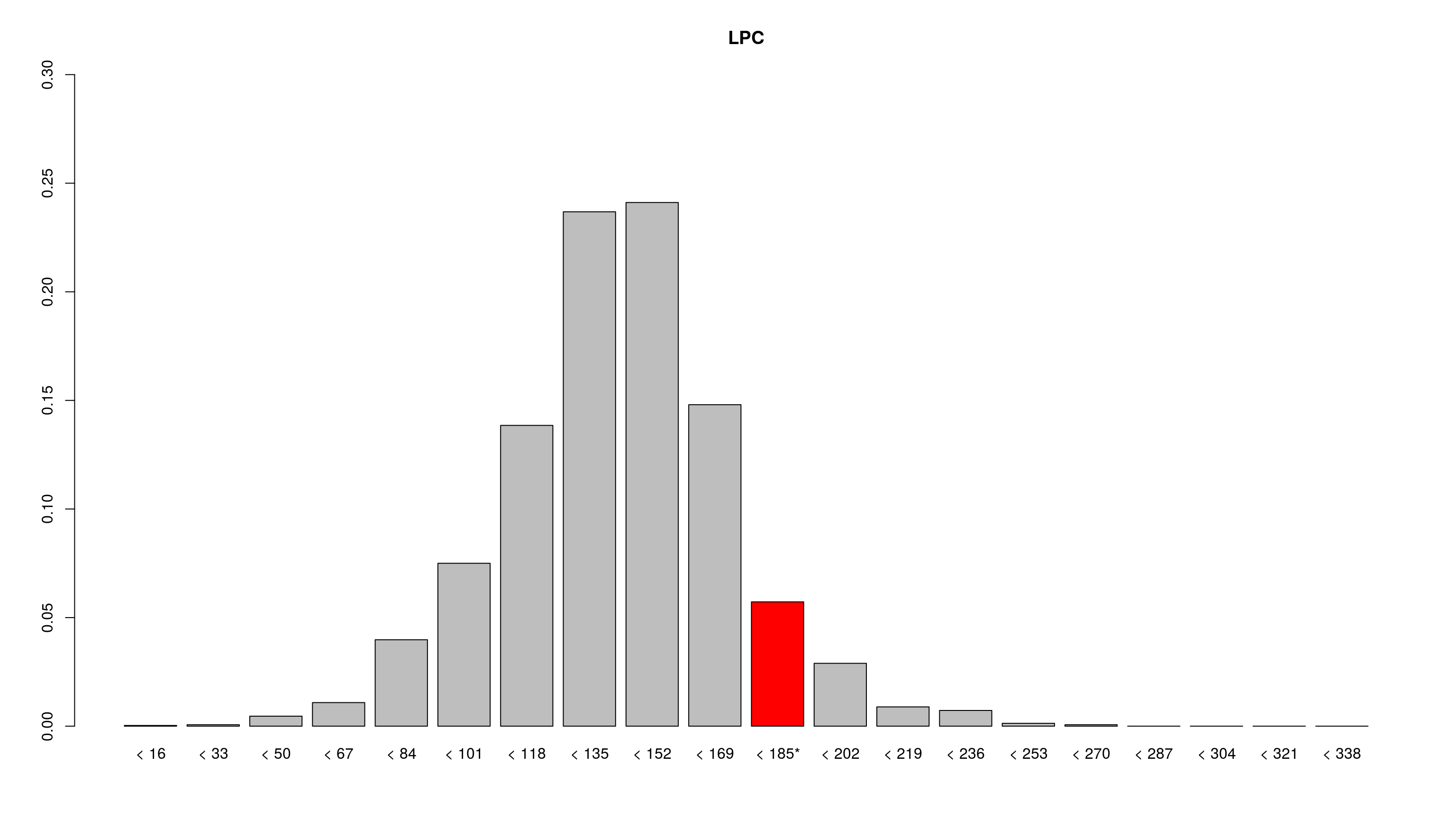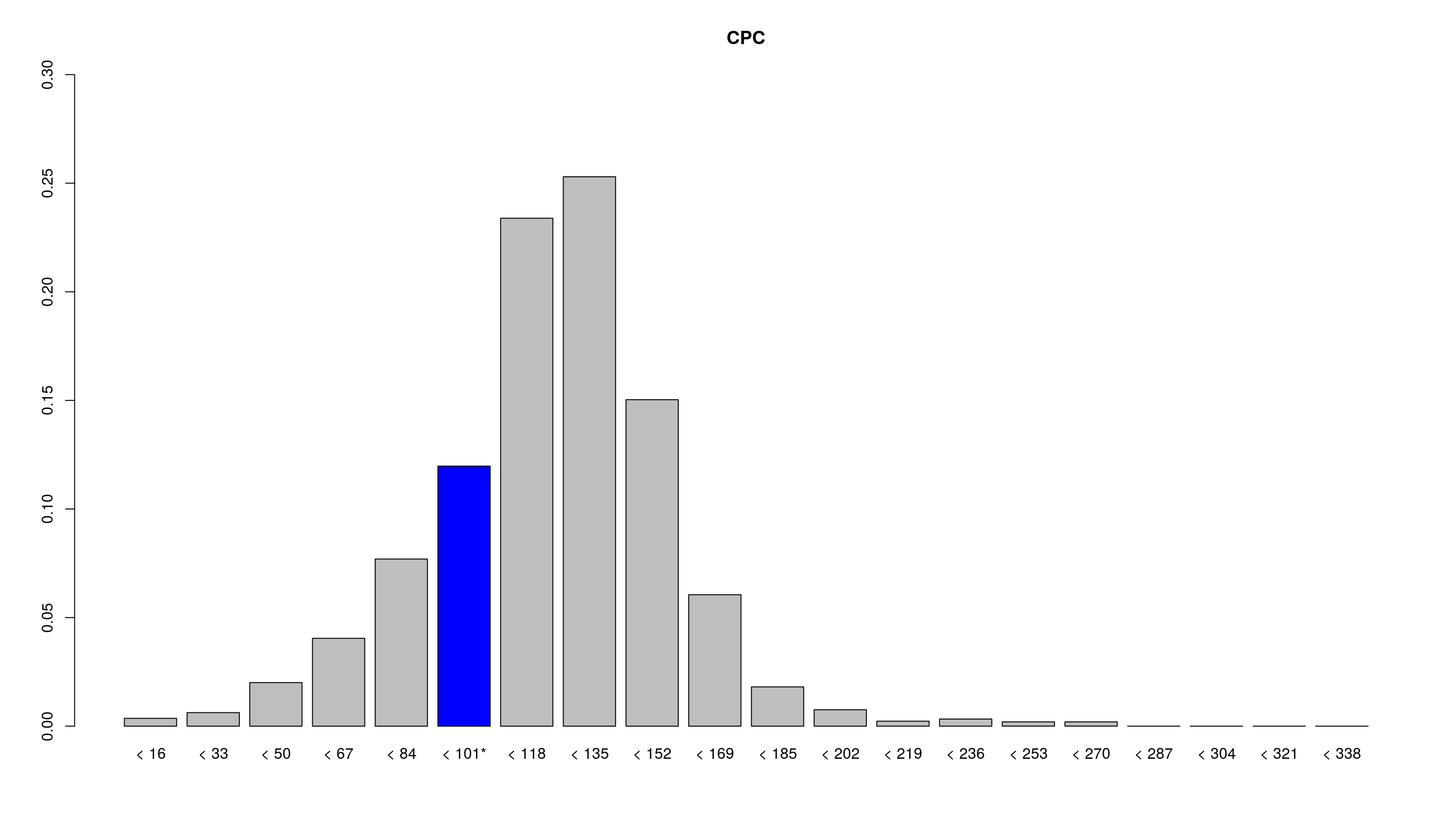The expectation as calculated from our forecaster’s subjective probability distributions and actual results for the combined subjective probability distributions for the NDP, LPC and CPC are reported in the table below. The final seat projection from CBC’s Poll Tracker and ThreeHundredEight.com are also provided for reference.

Party Expectation CBC6 3087 Actual outcome Difference
NDP 88 66 56 44 -44
LPC 134 146 154 184 50
CPC 116 118 120 99 -17

The LPC result was the most surprising, followed closely by the NDP result. The number of seats won by the CPC was the least surprising.

## Brier scores

Brier scores were calculated for provided subjective probability distributions for forecasts of the number of seats to be won by the NDP, LPC and CPC, and an overall Brier score was calcualted for each predictor as well, by taking the mean of the scores for each party’s seat prediction. The mean Brier score for all predictors was 0.0545, (95% confidence interval 0.0527–0.0562). Forecaster’s Brier scores are plotted in a histogram below, with the mean as a vertical purple line. For reference, because of the constraints placed on the forecasters (20 bins with a maximum of 50% certainty in one bin and a requirement that all chips be used), the worst possible prediction would receive a score of 0.075 (marked with a red vertical line) and the best possible prediction would receive a score of 0.013 (marked with a green vertical line).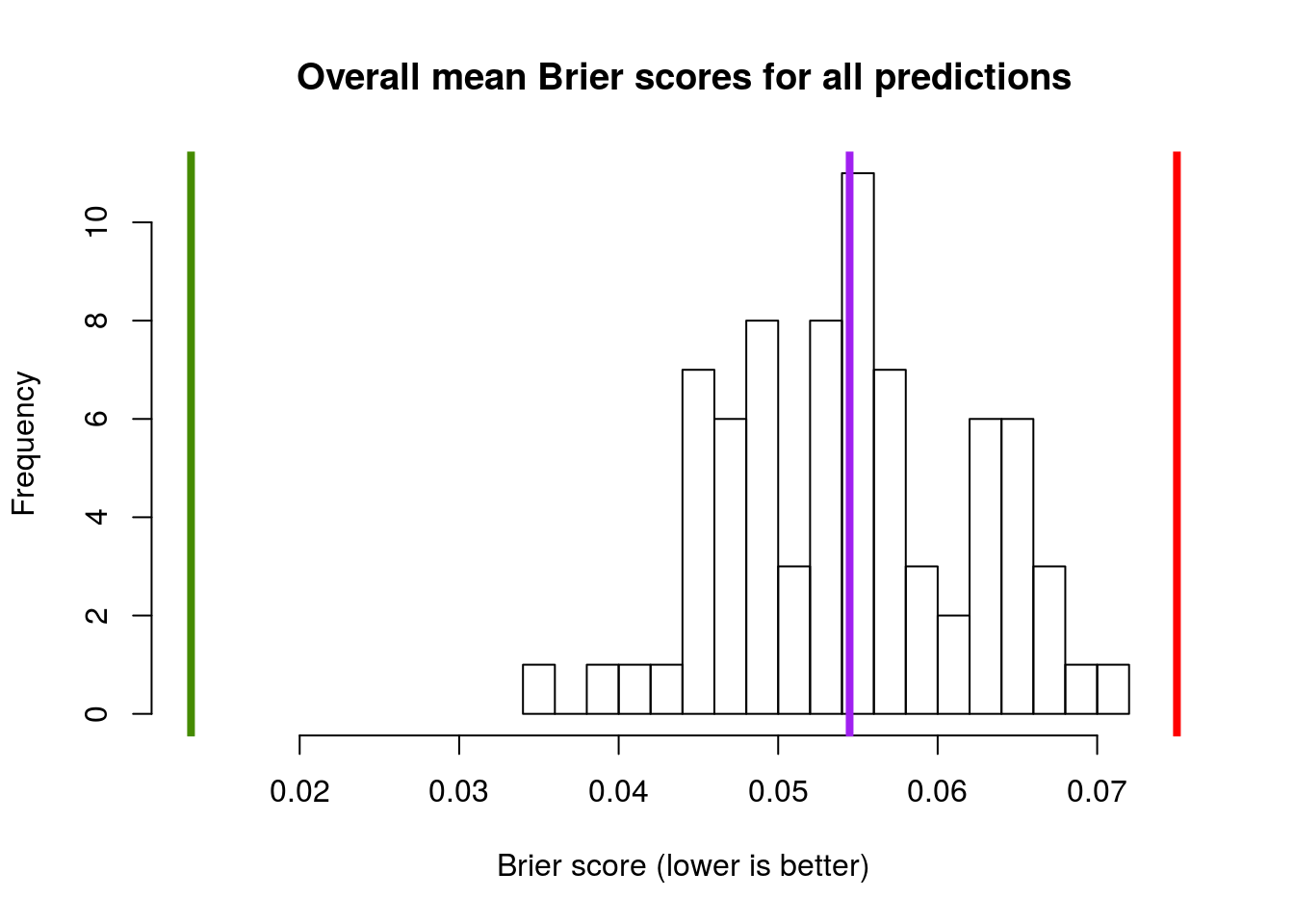The following is a box plot for Brier scores of forecasts of the number of seats in the House of Commons for the NDP, LPC and CPC. The mean Brier score for forecasts of the NDP was 0.0558 (95% confidence interval 0.0536–0.0581), 0.0571 (0.0547–0.0594) for the LPC and 0.0506 (0.0479–0.0532) for the CPC. The number of seats won by the LPC was more surprising than the second most surprising result, the seat count for the NDP, but not significantly so. The result for the CPC was least surprising, and significantly so.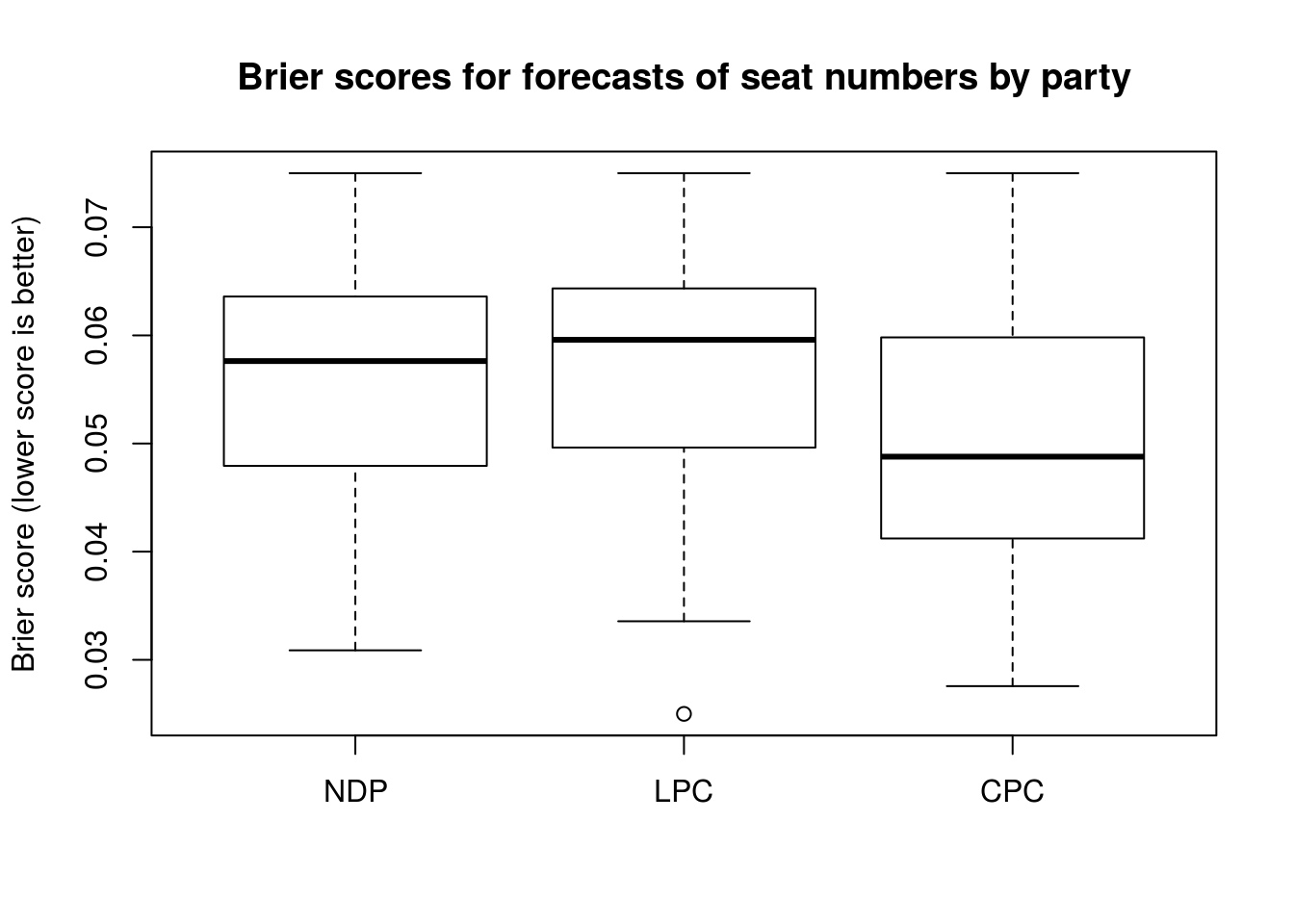## Forecasts of a Liberal majority

We summed the subjective probabilities assigned to the ranges of seat outcomes that corresponded to a LPC majority (namely, all columns representing outcomes of 170 seats or more). A histogram of the combined subjective probability of a Liberal majority outcome has been plotted below. Only 3 forecasters out of 76 forecasted Liberal majority with a certainty greater than 50%. In total, 38 out of 76 forecasters judged that there was a 0% probability of electoral outcomes that included a Liberal majority.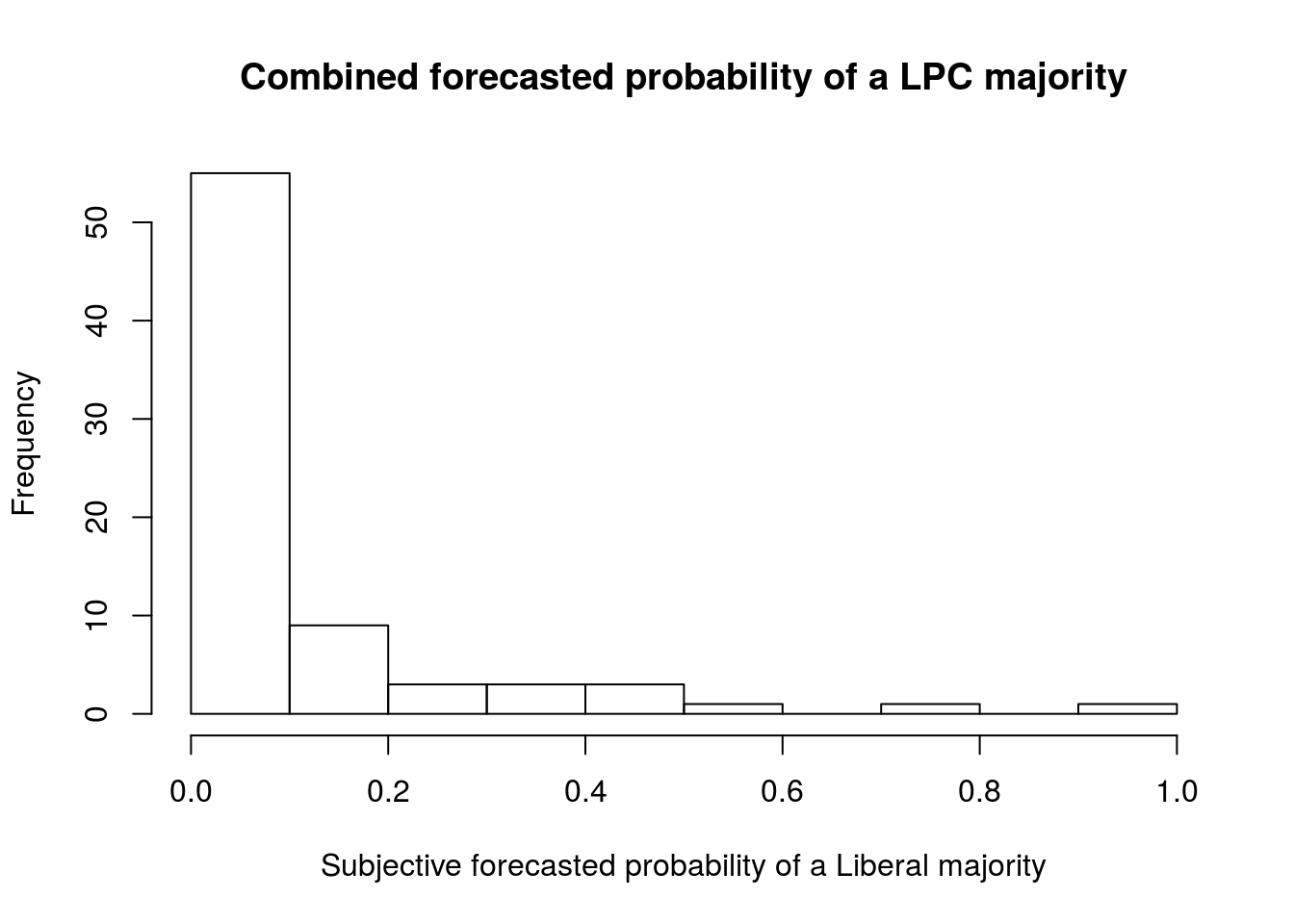## Comparison of Brier scores for supporters and non-supporters of each of the political parties

The mean Brier score for predictions of the NDP seat count among NDP supporters was 0.0568 (95% confidence interval 0.0529–0.0607), while the mean Brier score for predictions of the NDP seat count among those who did not identify as NDP supporters was 0.0553 (0.0525–0.0582).

The mean Brier score for predictions of the LPC seat count among LPC supporters was 0.0567 (0.0505–0.0629), while the mean Brier score for predictions of the LPC seat count among those who did not identify as LPC supporters was 0.0571 (0.0545–0.0597).

The mean Brier score for predictions of the CPC seat count among CPC supporters was 0.063 (0.000–0.127), while the mean Brier score for predictions of the CPC seat count among those who did not identify as CPC supporters was 0.0502 (0.0476–0.0529).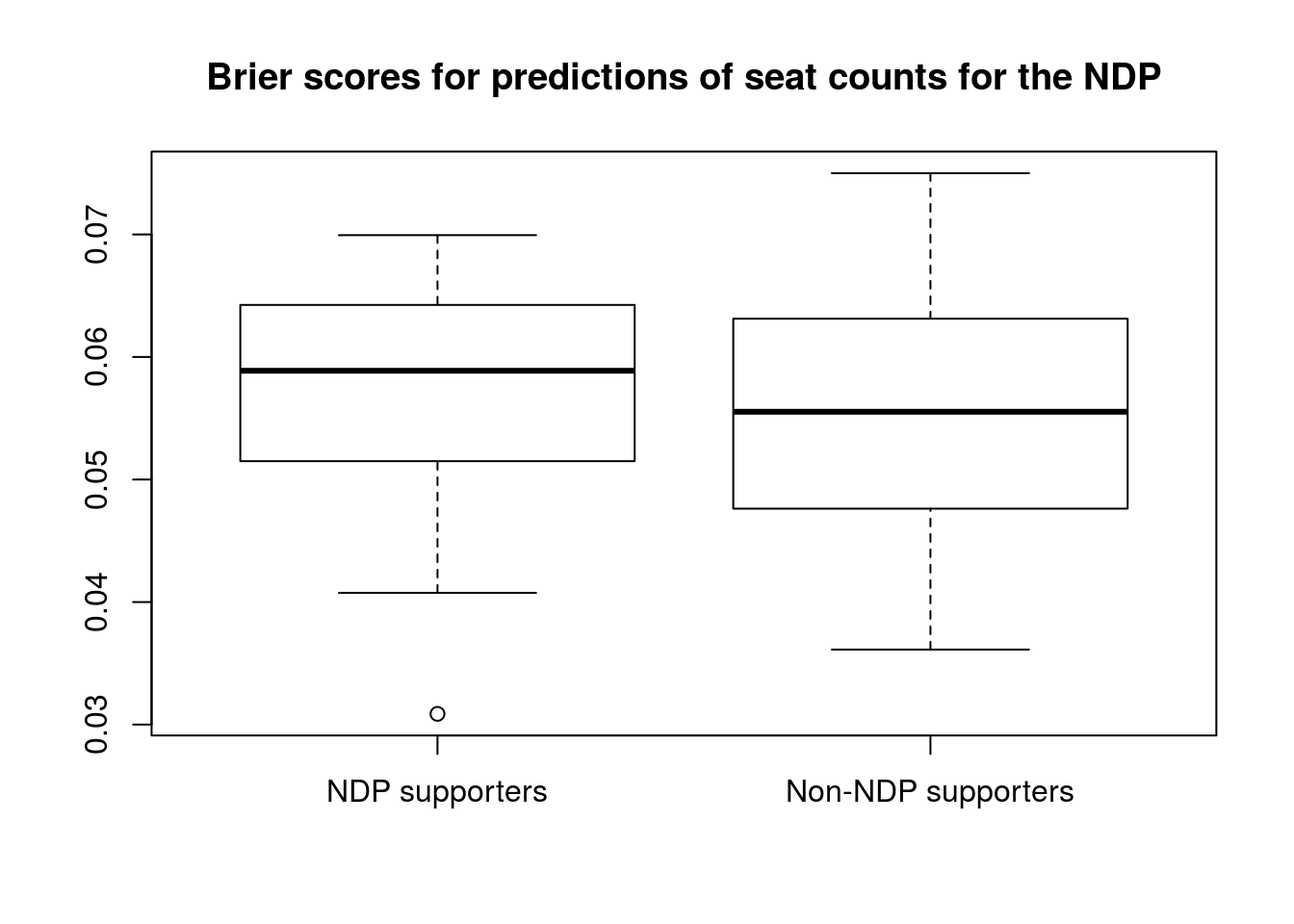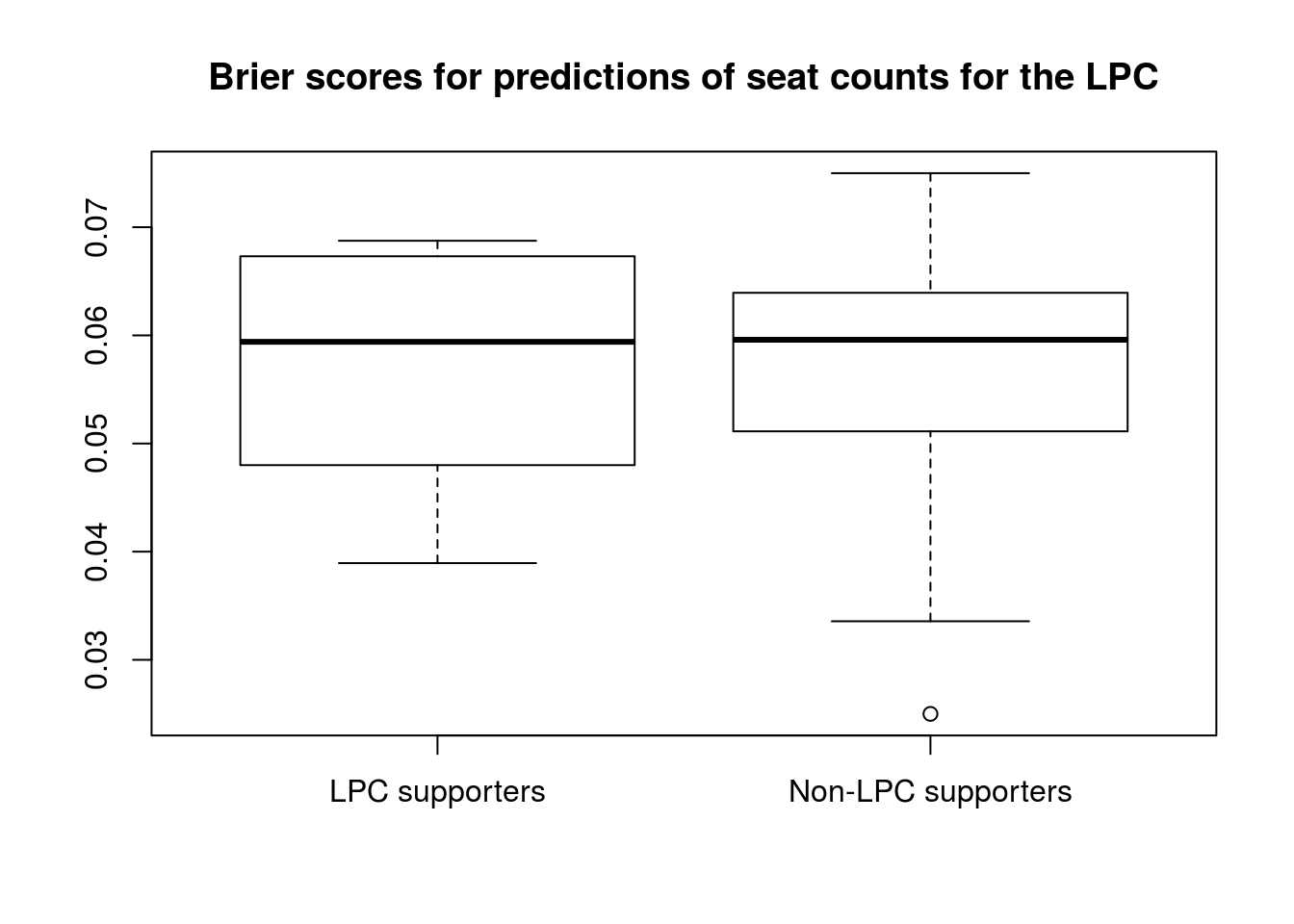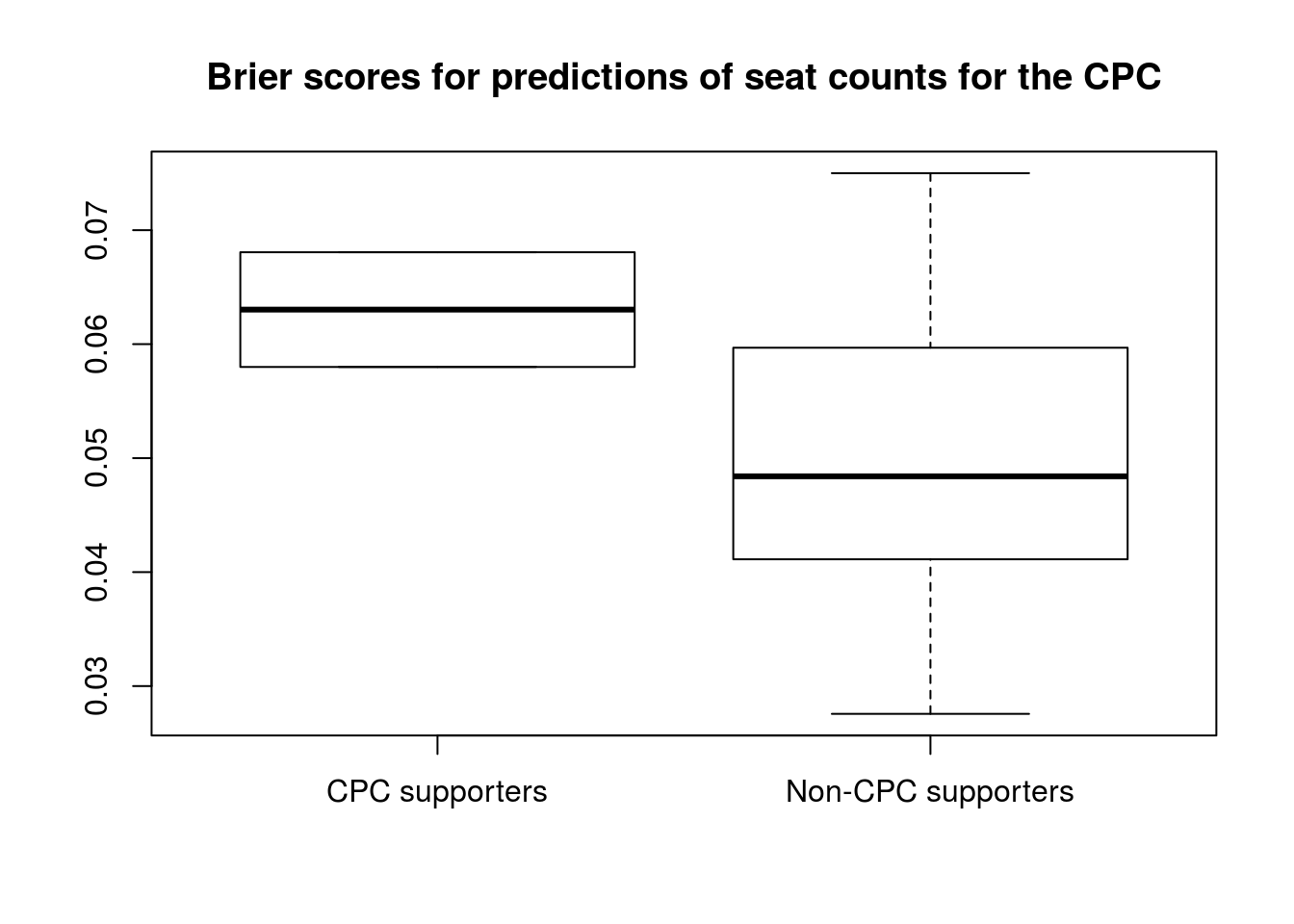## Party involvement

To test the relationship between self-reported level of party involvement (0–10, where 0 is “none” and 10 is “party leader”) and Brier score using a linear regression model. We considered only forecasters whose level of party involvement was greater than 0 ($$n=19$$), since a level of 0 could mean either “no involvement” or “decline to answer.” There was a trend toward worse predictions among forecasters with higher levels of party involvement, but this was not statistically significant ($$p = 0.48$$).